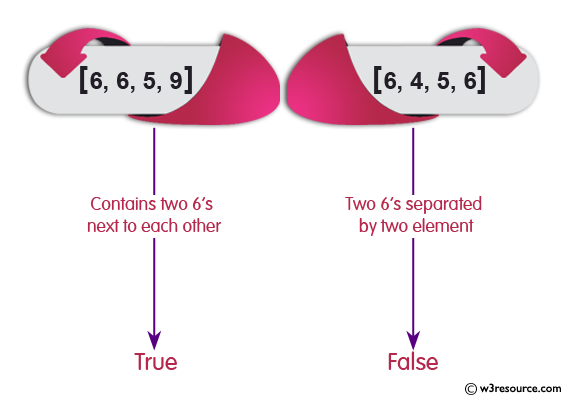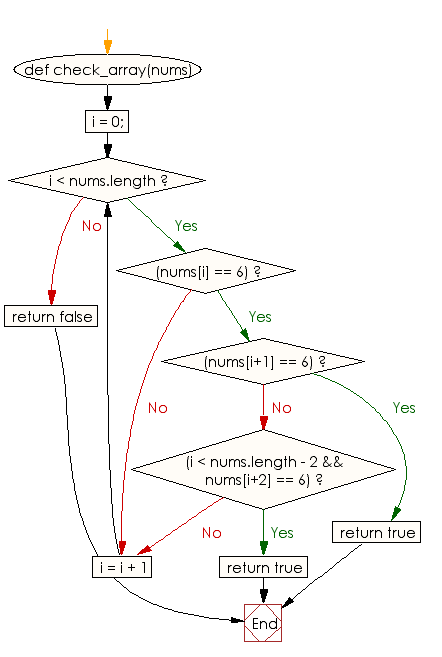﻿ Ruby Array exercises: Check whether a given array of integers contains two 6's next to each other, or there are two 6's separated by one element - w3resource# Ruby Array Exercises: Check whether a given array of integers contains two 6's next to each other, or there are two 6's separated by one element

## Ruby Array: Exercise-39 with Solution

Write a Ruby program to check whether a given array of integers contains two 6's next to each other, or there are two 6's separated by one element, such as {6, 2, 6}.Ruby Code:

``````def check_array(nums)
i = 0;
while i < nums.length
if(nums[i] == 6)
if(nums[i+1] == 6)
return true
elsif(i < nums.length - 2 && nums[i+2] == 6)
return true
end
end
i = i + 1
end
return false
end
print check_array([6, 3, 6, 5]),"\n"
print check_array([6, 6, 5, 9]),"\n"
print check_array([6, 4, 5, 6]),"\n"
``````

Output:

```true
true
false
```

Flowchart:Ruby Code Editor: